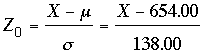SEARCH HOMEMath Central Quandaries & QueriesQuestion from Shawn, a parent: For a normal distribution of u=654.00 and o=138.00. What is the percentile rank for X=426?Shawn,

You have the normal distribution with mean μ = 654.00 and standard deviation σ = 138.00. Convert to the standard normal distribution usingUse the value of Z obtained and your standard normal table to find

Pr(Z < Zo)

The percentile rank is this probability, expressed as a percentage.

I hope this helps,
HarleyMath Central is supported by the University of Regina and The Pacific Institute for the Mathematical Sciences.